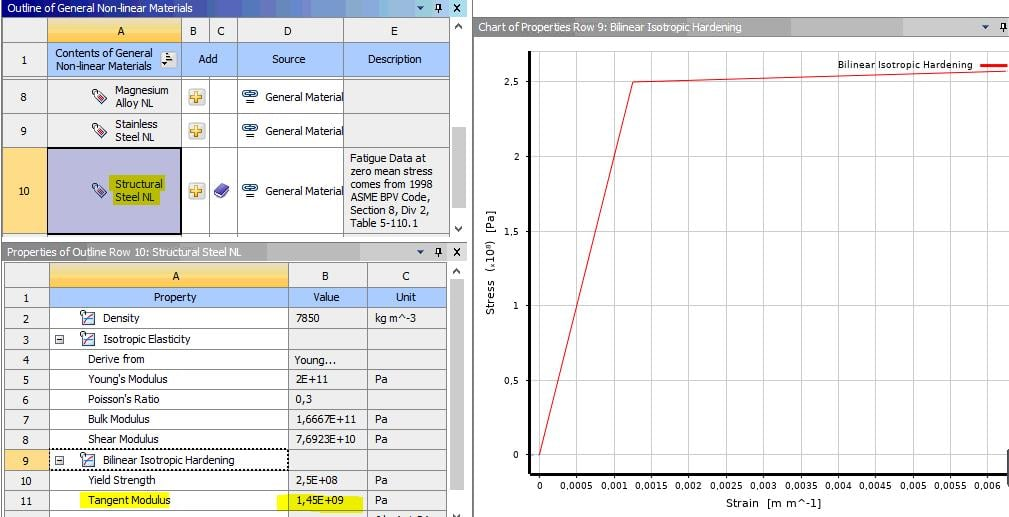## General Mechanical

•Cri1994
Subscriber

Hi everyone,

I'm doing a non-linear analysis and I'm using a Bilinear model. I have to insert the Yeld stress of material and the tangent modulus.

Where can I find the Tangent modulus of materials?

Best regards

Cristiano

•peteroznewman
Subscriber

A simple bilinear material model called Elastic Perfectly Plastic (EPP) is often used in engineering analysis. The Tangent Modulus is zero. This material model usually provides conservative predictions of failure. That means if you simulate a safety test and pass with this material model, it is highly likely that a physical test will pass since the real material has some non-zero tangent modulus that can carry more load than the EPP material model.

•piknockyou
Subscriber

Thanks. But how do you calculate the Tangent Modulus for a simplified analysis approximately?

I can't find anything about it.

ANSYS has this generic material in the material library with a Tangent Modulus of 1450 MPa.

How is it calculated?•SaiD
Ansys Employee

Hi,

The Tangent Modulus for a material with Bilinear Hardening (such as the one here) is the slope of the curve beyond the yield stress. In the plot above, the first line with a high slope has a slope of 2E11 Pa (i.e. slope is equal to the Young's Modulus). The second line which has a much smaller slope (and appears almost flat) has a slope equal to the Tangent Modulus i.e. its slop is 1.45E9 Pa.

Hope this helps,

Sai

•shaghayegh.nazar
Subscriber

Hi every one

I want to model static crack growth under elastic-plastic fracture mechanics, I choose bilinear for first  step ( fracture-pre meshed crack-smart crack ( static)) and gave me an error can you guide me